# Quiz 14: Replacement Decisions

Business

In economics, the opportunity cost refers to the benefits given up by an individual or business when an alternative is chosen over another. Sunk cost refers to the cost incurred in past and it is not affected by the future investments. a. For replacement analysis, the relevant cost information is original cost, market value, and trade-in allowance. The cost of the machine in option 1 is \$12,000 and it can be sold for \$2,000. In option 1, the sunk cost for the old machine is the cost incurred and cannot be recovered. The sunk cost for the old machine can be calculated as follows:Hence, the sunk cost for the old machine tools recognized in replacement analysis is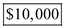. b. According to the opportunity cost approach, the net proceeds from the sale is the opportunity cost of keeping the defender. In simple words, the salvage value is the amount of investment required to keep the defender. The salvage of the old machine tool now is \$2,000 and if it is used for the next three years then the salvage value will be zero. According to the opportunity cost approach, the opportunity cost of keeping the old machine tool now is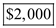. c. The net incremental benefit or loss in present value for replacing old machine tool with new machine tool at an interest rate of 15% can be computed from the difference of the present value of each machine tool. Calculate the present value of the old machine tool as follows:Thus, the present value of the old machine tool is \$24,832. Calculate the present value of a new machine tool as follows:Thus, the present value of the new machine tool is \$18,562.1. The above calculated present worth shows that the annual difference of \$6,269.9 is in favour of the new machine tool. Thus, the old machine should replace with a new machine tool. Hence, the net incremental benefit in present value related to replacing old machine tool with new machine tool is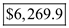.

Annual Equivalent Cost (AEC) method is used when the costs are only involved in the particular project. In this method, the revenue earned must recover two types of cost that is capital cost and operating cost. A conveyor system was installed in the manufacturing plant with the salvage value of \$3,000. The estimated market values and operating costs are given for the three years. The MARR is given to be 18%. (a) It is required to determine the remaining economic service life of the old machine. It is calculated with the appropriate formula in the spreadsheet given below: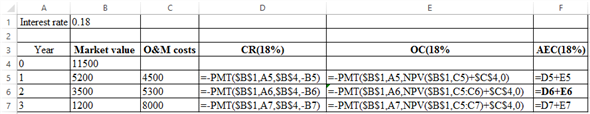The corresponding values are: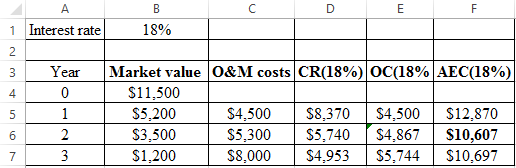The minimum AEC value is found to be \$10,607. Hence, the remaining economic life of the old machine is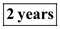. (b) It is required to find the decision whether replacing the defender or not. The Annual Equivalent Cost of the defender is calculated to be \$10,607. The Annual Equivalent Cost of the challenger is calculated with the appropriate formula as shown below:As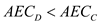then it is advisable not to replace the defender now. (c) It is required to find the right time for replacing the defender. First, it is required to find the marginal cost by keeping the defender for one more year.So, now it would be better to replace the defender at the end of. (d) In order to reach the conclusion given in part c, it is required to have the future cost of the challenger to be same.

a) The first part of the problem that one would need to get answer for here has to do with finding the sunk cost for the project. Remember that a sunk cost is one that has already occurred in the past and cannot be recouped now. In other words, the \$15,000.00 price that was paid three (3) years ago is already long and gone. However, the residual salvage value of \$6,000.00 is not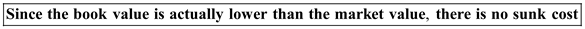. On a side bar, though, another way to view it is the difference between the initial cost of the asset and the residual salvage value. Though this amount of \$9,000.00 can be viewed as a sunk cost as well, this should not be considered when doing a replacement analysis. b) The next question that is on the board asks one to determine the opportunity cost for the problem at hand. Remember that the opportunity cost is an economical value that one would sacrifice when choosing an alternative option that may be available or will become available. The best way to handle a problem like this is to create a table which one can see the numbers as they incrementally change through each piece of the opportunity cost process. This is shown below Table 1.1 for one to see: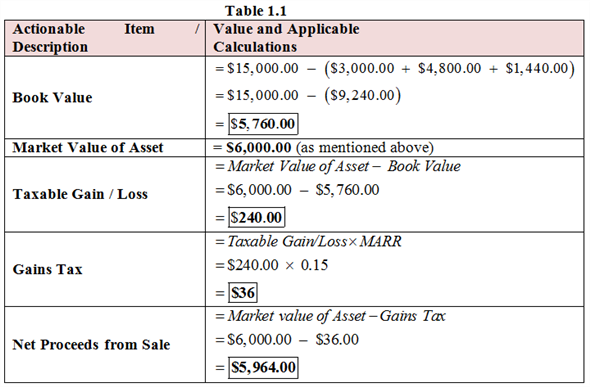Thus, the opportunity cost applicable for not replacing the old vehicle now, which is \$5,964.00, can be viewed as an investment for keeping the other one running. Also, note that since the market value is less than the original purchase cost, there are no actual capital gains applicable here. Thus, the taxable gain in the amount of \$96.00 is considered an ordinary gain. c) The third part of the problem one would need to calculate here has to do with finding the annual equivalent worth of owning the existing forklift truck for a period of two (2) more years. The way that one would get the answer here is in two (2) phases. The first one is to determine the present worth at a specific interest rate provided. The formula and calculations are shown below for one to see: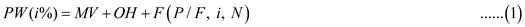Where, P = present value of the sum of money, A = the annual payment applicable to the situation here, MV = the market value (which is negative ), OH = the overhaul costs applicable to the situation, i = interest rate (which is unknown in this case), and N = number of terms that the money is for. All one would need to do here is to apply the numbers that we got from the cash flow series at the top of the problem to get the applicable answer here:The next step that one has to do here is to mosey on back to Appendix B in the latter part of the textbook. In this appendix, there are a number of factor value tables that one will need to use and become familiar with very quickly. In this situation, one would want to look at the second column for the factor value here for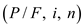. We continue below with the applicable factor values applied to the equation:Thus, the present worth of the existing machine in question at an interest rate of 15.00% is approximately -\$10,486.85. We now need to turn our attention to the annual equivalence for the asset in focus. Since we need this on a consistent scale, we would need to apply the second formula of the pot to get the proper answer we need here. Note that the value of P (which is what we got above) will always be positive in this question, Thus, this is shown below for one to see and its corresponding calculations: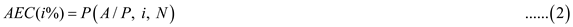Where, P = present value of the sum of money, F = future value of the sum of money, A = the annual payment applicable to the situation here, i = interest rate (which is unknown in this case), and N = number of terms that the money is for. All one would need to do here is to apply the numbers that we got from the cash flow series at the top of the problem to get the applicable answer here: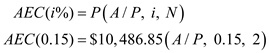The next step that one has to do here is to mosey on back to Appendix B in the latter part of the textbook. In this appendix, there are a number of factor value tables that one will need to use and become familiar with very quickly. In this situation, one would want to look at the sixth column for the factor value here for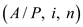. We continue below with the applicable factor values applied to the equation:Thus, the annual equivalent costs at an interest rate of 15.00% are approximately \$6,450.56 for the company keeping the forklift truck for two (2) more years. d) The final part of the problem one would need to calculate here has to do with finding the annual equivalent worth of owning the existing forklift truck for a period of five (5) more years. Note that this would be calculated much in the same way as we have done it above with the exception that each of the five (5) annual operating and maintenance costs must be factored in separately. In addition to that, one would not want to forget the major overhaul in the fifth year of \$5,000.00. To shorten the problem solving and provide an answer in less time, the present worth of the five (5) year time frame comes out to approximately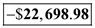while the annual equivalent costs (the crux of this question) is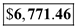.

There is no answer for this question